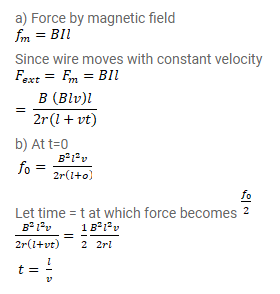# Consider the situation of the previous problem (a) Calculate

Question:

Consider the situation of the previous problem (a) Calculate the force needed to keep the sliding wire moving with a constant velocity $v .$ (b) If the force needed just after $\mathrm{t}=0$ is $F_{0}$, find the time at which the time at which the force needed will be $F_{0} / 2$.

Solution: